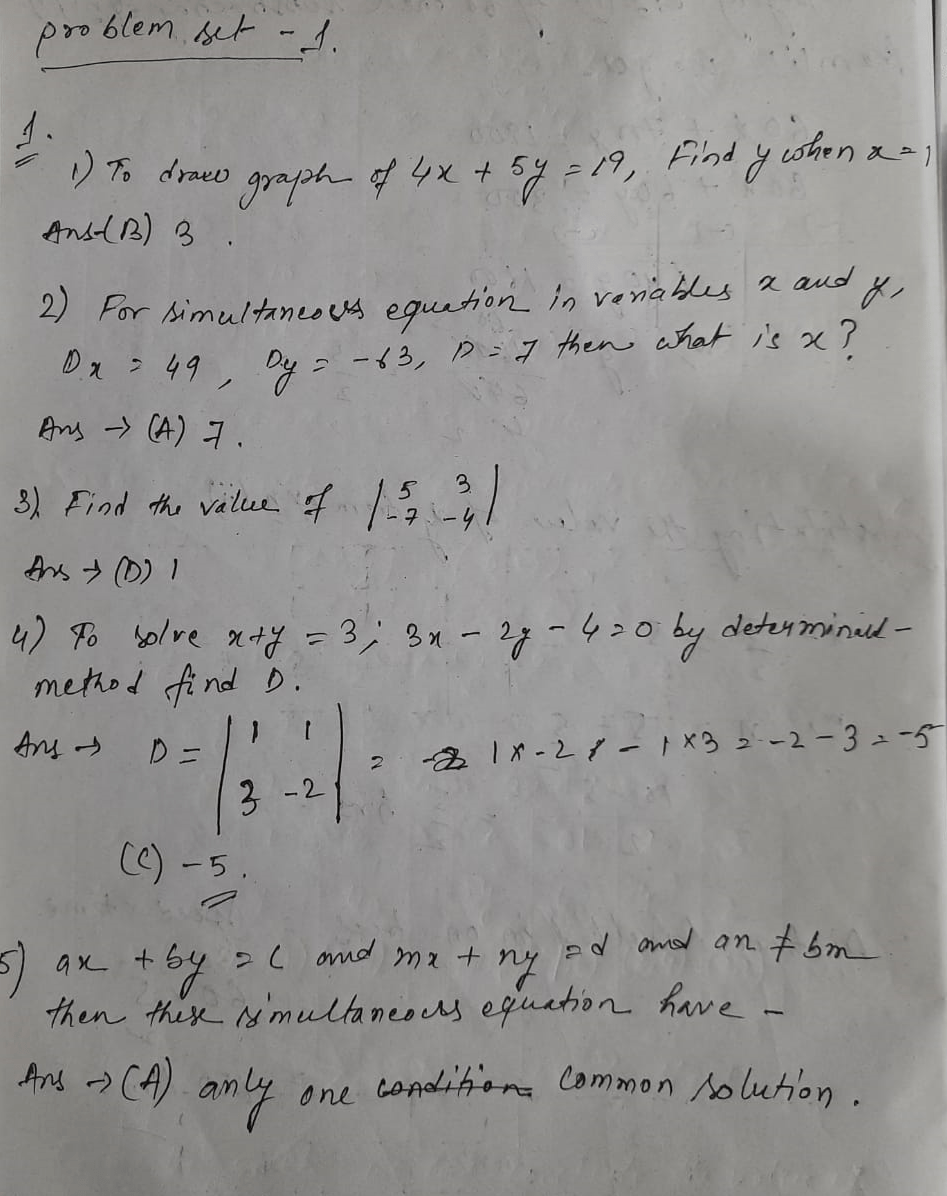# Maharashtra Board Class 10 Math Part 1 Chapter 1 Problem set 1 Linear Equations in Two Variables Solution

## Maharashtra Board Class 10 Math Part 1 Solution Chapter 1 Problem set 1 – Linear Equations in Two Variables

Balbharati Maharashtra Board Class 10 Math Part 1 Solution Chapter 1: Linear Equations in Two Variables. Marathi or Math Part 1 Medium Students of Class 10 get here Linear Equations in Two Variables full Problem set Solution.

 Std Maharashtra Class 10 Subject Math Part 1 Solution Chapter No- 1 Chapter Name- Linear Equations in Two Variables Problem set 1

### Problem set – 1Here is your solution of Maharashtra Board Class 10 Math Part 1 Solution Chapter 1 Problem set 1 – Linear Equations in Two Variables

Dear Student, I appreciate your efforts and hard work that you all had put in. Thank you for being concerned with us and I wish you for your continued success.

Updated: October 5, 2021 — 11:24 am